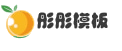# 自己动手添加一个函数 实现任意字段调用

2021-07-28 0 5,633

function table(\$table, \$field, \$id)
{
global \$dsql;
\$primarys = array();
\$table = strpos(\$table, '#@_') === false?(strpos(\$table, 'dede_') ===false?''.\$table:str_replace('dede_','',\$table)):\$table;
\$dsql -> Execute("me","SHOW COLUMNS FROM `\$table`");
while (\$r = \$dsql->GetArray())
{
if(\$r['Key'] == 'PRI') \$primarys[] = \$r['Field'];
}
if(!empty(\$primarys))
{
\$primary = \$primarys;
\$result = \$dsql -> GetOne("SELECT `\$field` FROM `\$table` WHERE `\$primary`= \$id");
}
return isset(\$result[\$field])?\$result[\$field]:'';
}

{dede:标记 function='table("要调用的表名","要调用的字段",@me)'/}

[field:字段 function=table(“要调用的表名","要调用的字段",@me)/]

{dede:arclist row='10' titlelen='24'}
{/dede:arclist}• 模板团承诺演示效果图均和网站95%一样，并且我们的模板和数据都没有留后门，请放心使用。查看详情
• 因为模板具有可复制性和可传播性，模板一旦发货，非质量问题，不支持退换；所以务必请您一定看清楚后再购买。查看详情

### 相关文章3个月前 2,7863个月前 2,8733个月前 3,7103个月前 3,465

• 0 +

访问总数

• 0 +

会员总数

• 0 +

资源总数

• 0 +

今日发布

• 0 +

本周发布

• 0 +

运行天数